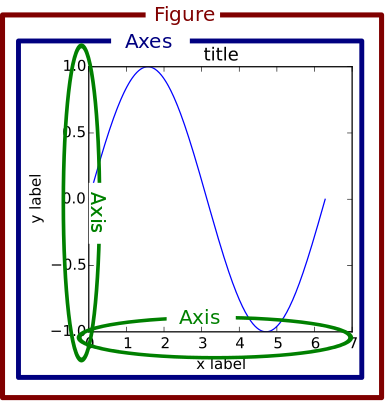# Clarification of Axes and Fig

Can I get some clarification on when to use `plt.` and when do we use `ax.set_`?

I am also unsure as to what exactly does `ax, fig = plt.subplots()` mean.

From off the top of my head, plt (a named representation of matplot.pyplot) allows you to create plots in python
So it is common to run
`import matplotlib.pyplot as plt`

plot.subplots allows you create a figure and set of subplots, and you can arrange each whichever way you want in the figure

I also presume you’re asking for the difference between plt.xxx() (for example) and ax.set_xxx()
The ax methods are object oriented setter methods that were later developed by matplotlib (the plt.xxx() are older versions of the same thing) - https://matplotlib.org/3.2.1/tutorials/introductory/lifecycle.html should explain some more

When you run `fig, ax = plt.subpolots()` you are instantiating two objects. The figure object and the axes object.

A Figure object is the outermost container for a `matplotlib` graphic, which can contain multiple Axes objects. It works like this:So every time you use a method on the ax object, like `ax.set_title()` you are performing an action on the axes object inside the figure object. You can create as many axes inside a figure as you want using the `nrows` and `ncols` on `plt.subplots()`

You can read more about on the article below (from where I took some resources to answer your question) and from the official documentation.

2 Likes

I think the diagram makes it easier to understand. Thanks! Will check out the link to get a better picture.

1 Like